# River

From the observatory 11 m high and 24 m from the river bank, river width appears in the visual angle φ = 13°. Calculate width of the river.

Result

y =  29.5 m

#### Solution:

$h=11 \ \text{m} \ \\ x=24 \ \text{m} \ \\ A=13 ^\circ \rightarrow\ \text{rad}=13 ^\circ \cdot \ \dfrac{ \pi }{ 180 } \ =13 ^\circ \cdot \ \dfrac{ 3.1415926 }{ 180 } \ =0.22689 \ \\ B=\arctan (x/h)=\arctan (24/11) \doteq 1.141 \ \\ C=A+B=0.2269+1.141 \doteq 1.3679 \ \text{rad} \ \\ c=180/\pi \cdot \ C=180/3.1416 \cdot \ 1.3679 \doteq 78.3764 \ ^\circ \ \\ \tan C=(x+y)/h \ \\ y=h \cdot \ \tan(C)-x=11 \cdot \ \tan(1.3679)-24 \doteq 29.4762 \doteq 29.5 \ \text{m}$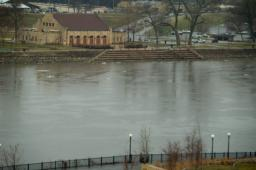Our examples were largely sent or created by pupils and students themselves. Therefore, we would be pleased if you could send us any errors you found, spelling mistakes, or rephasing the example. Thank you!

Leave us a comment of this math problem and its solution (i.e. if it is still somewhat unclear...):Be the first to comment!Tips to related online calculators
Do you have a linear equation or system of equations and looking for its solution? Or do you have quadratic equation?
Do you want to convert length units?
Pythagorean theorem is the base for the right triangle calculator.

## Next similar math problems:

1. The rescue helicopterThe rescue helicopter is above the landing site at a height of 180m. The site of the rescue operation can be seen from here at a depth angle of 52° 40 '. How far will the helicopter land from the rescue site?
2. The angle of viewDetermine the angle of view at which the observer sees a rod 16 m long when it is 18 m from one end and 27 m from the other.
3. A kite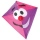Children have a kite on an 80m long rope, which floats above a place 25m from the place where children stand. How high is the dragon floating above the terrain?
4. An equilateralAn equilateral triangle is inscribed in a square of side 1 unit long so that it has one common vertex with the square. What is the area of the inscribed triangle?
5. Interior anglesCalculate the interior angles of a triangle that are in the ratio 2: 3: 4.
6. The basesThe bases of the isosceles trapezoid ABCD have lengths of 10 cm and 6 cm. Its arms form an angle α = 50˚ with a longer base. Calculate the circumference and content of the ABCD trapezoid.
7. The angles ratioThe angles in the ABC triangle are in the ratio 1: 2: 3. find the sizes of the angles and determine what kind of a triangle it is.
8. The right triangleThe right triangle ABC has a leg a = 36 cm and an area S = 540 cm2. Calculate the length of the leg b and the median t2 to side b.
9. In aIn a triangle, the aspect ratio a: c is 3: 2 and a: b 5: 4. The perimeter of the triangle is 74cm. Calculate the lengths of the individual sides.
10. Annulus from triangleCalculate the content of the area bounded by a circle circumscribed and a circle inscribed by a triangle with sides a = 25mm, b = 29mm, c = 36mm
11. Height to the baseThe triangle area is 35 cm ^ 2. The size of the base is 10 cm. Find the length of height to the base.
12. Black diamond run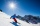Taleah is skiing down a black diamond run. She begins skiing at the top of a ski trail whose elevation is about 8625 feet. The ski run ends toward the base of the mountain at 3800 feet. The horizontal distance between these two points is about 4775 feet.
13. RT triangle and heightCalculate the remaining sides of the right triangle if we know side b = 4 cm long and height to side c h = 2.4 cm.
14. Clock handsCalculate the internal angles of a triangle whose vertices lie on the clock's 2, 6 and 11 hours.
15. The angles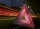The angles in the triangle are in the ratio 12: 15: 9. Find the angles.
16. The triangles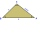The triangles KLM and ABC are given, which are similar to each other. Calculate the lengths of the remaining sides of the triangle KLM, if the lengths of the sides are a = 7 b = 5.6 c = 4.9 k = 5
17. Similarity of two trianglesThe KLM triangle has a side length of k = 6.3cm, l = 8.1cm, m = 11.1cm. The triangle XYZ has a side length of x = 8.4cm, y = 10.8cm, z = 14.8cm. Are triangle KLM and XYZ similar? (write 0 if not, if yes, find and write the coefficient of a similarity)